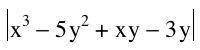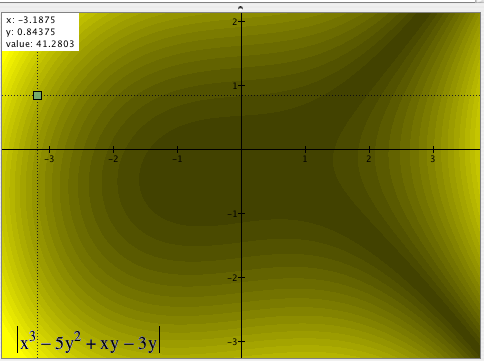What follows is a reprise of our discussion in class. It ends with a table giving the first few steps of the activity in which you engaged. The aim of this assigmnent is that you re-create the logic of that activity, so that you not only re-create the activity itself, but also create a justification of it that makes sense to you. NOTE: The expression used here differs from the one we used in class. This is to highlight the logic of the activity instead of focusing you on replicating the activity.

# Inequalities

Students often have a very hard time understanding inequalities and their solution sets. They often approach inequalities as if the idea is unrelated to everything they have done. This activity suggests one way to approach the idea of inequality so that what is conventionally proposed as an out-of-the-blue procedure instead emerges as a natural response to very sensible questions.

For them to understand the idea of inequalities and their solution sets, they must have a particular understanding of equations and variables.

## Equations

How students understand equations is either the biggest obstacle or the biggest aid to their coherent understanding of inequalities.

 3x2+2x-7 = 4 3x2+2x-7 < 4 UNDERSTANDING OF EQUATIONS THAT POSES AN OBSTACLE IF students think of this equation as "the answer" being a number that hides behind the letter "x", and that his or her responsibility is to figure out what that number is, THEN they think the implied question is, "What is x?"they are thinking as if x is a constant, not a variablethey are thinking that the statement is offered as a true statement, and therefore they cannot replace x with values that "don't work" AS A RESULT they will likely think of " 3x2+2x-7 < 4" in a number of confusing ways: there is supposed to be a number behind x (like an equation), but there is no one definite number there are lots of numbers hiding behind "x" and their job is to find them. inequalities have nothing in common with equations UNDERSTANDING OF EQUATIONS THAT IS SUPPORTIVE IF students think of this equation as an open sentence, a statement that is neither true nor false by itself, and becomes true or false as you replace x with various values, then they think the implied question is, "What values, when substituted for x, make the statement true?"they feel free to substitute any value for x they can see that the set of possible values is partitioned into two parts -- those values that make the statement true and those values that make the statement false AS A RESULT they will likely think of this statement more coherently: out of all the possible values that x can have, which ones make the statement true and which ones make the statement false?inequalities are just like equations

## Inequalities

The following activity can be used to introduce students to the idea of inequalities. It begins with inequalities in two variables rather than inequalities in one variable. This is because the added complexity actually increases the level of interest students feel.

### To do this activity you will need

• Graphing Calculator (3.0 or higher) from PacificTech.
• A clear overhead transparency sheet
• Scotch tape
• Transparency marker (erasable)

### The activity

Be sure to remind students of the meaning you already established for equations (see prior comments), the implied question, and what a solution set is.Click on the graph to get a GC file for this activity,

OR

• Type x3 - 5y2 + xy - 3y into Graphing Calculator (DO NOT press enter)
• Select Graph>Density Plot (i.e., in the Graph menu, select Density Plot
• Press Enter (or click Graph)

## Lesson Logic (for you to complete)

 Step Action Reason 1. Discuss with students that the expression at the page's top is simply a calculation rule. It says what to do with values of x and y once they are given. You might give them several values of each for them to calculate. You don't want students to think that you expect them to solve anything nor that you expect them to "know what x and y are". 2. Tell them to click anywhere on the graph (but not on an axis). Explain that where they clicked indicates a point in the coordinate plane, and that GC tells them the point's x-coordinate, its y-coordinate, and the value of the expression after substituting those values of x and y into it. Have them click several places so that they can see that this is indeed how it works. This familiarizes them with the program's interface and builds confidence that this complicated formula can be evaluated easily for whatever values of x and y they choose. 3. Ask students to use their markers to indicate 5 points on the screen that have coordinates that make the expression have a value less than 6. ... ... ... 4. (You complete the rest of this table) 5. 6. . . . . . . . . .